# Mechanical Engineering - Engineering Mechanics

### Exercise :: Engineering Mechanics - Section 6

21.

The cartesian equation of trajectory is(where u = Velocity of projection, α = Angle of projection, and x, y = Co-ordinates of any point on the trajectory after t seconds.)

 A.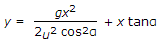B.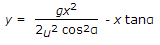C.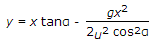D.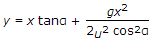Explanation:

No answer description available for this question. Let us discuss.

22.

In collision of elastic bodies, the coefficient of restitution is the ratio of the relative velocity after impact to the relative velocity before impact.

 A. True B. False

Explanation:

No answer description available for this question. Let us discuss.

23.

In a framed structure, as shown in the below figure, the forces in the members AB and BC are respectively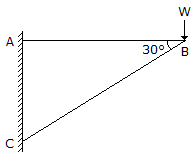A.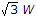(tensile) and 2W (compressive) B. 2W(tensile) and(compressive) C.(tensile) and(compressive) D. none of the above

Explanation:

No answer description available for this question. Let us discuss.

24.

The acceleration of a particle moving with simple harmonic motion is proportional to the displacement of the particle from the mean position.

 A. True B. False

Explanation:

No answer description available for this question. Let us discuss.

25.

The unit of moment of inertia of an area is

 A. kg-m2 B. kg-m-s2 C. kg/m2 D. m4